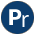#arithmetic.pl -- Extensible arithmetic

This module provides a portable partial replacement of SWI-Prolog's user-defined arithmetic (evaluable) functions. It defines the compatibility directive arithmetic_function/1 and support for both runtime and compile-time evaluation of expressions that are a mixture between Prolog predicates used as functions and built-in evaluable terms.

arithmetic_function(:NameArity) is det
Declare a predicate as an arithmetic function.
deprecated
- This function provides a partial work around for pure Prolog user-defined arithmetic functions that has been dropped in SWI-Prolog 5.11.23. Notably, it only deals with expression know at compile time.
eval_clause(+Term, -Clause) is det[private]
Clause is a clause for evaluating the arithmetic expression Term.
arithmetic_expression_value(:Expression, -Result) is det
True when Result unifies with the arithmetic result of evaluating Expression.
evaluable(F) is semidet[private]
True if F and all its subterms are evaluable terms or variables.
tidy(+GoalIn, -GoalOut)[private]
Cleanup the output from expand_function/3.